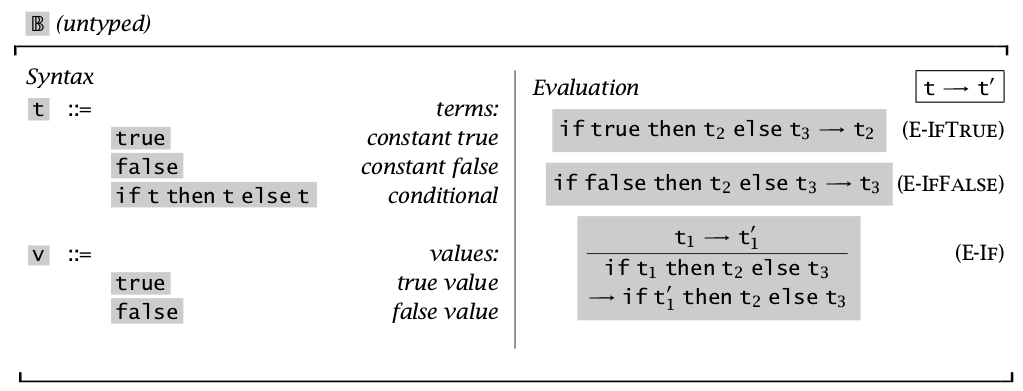# Prove determinacy for a small language

Recently I started reading the book Types and Programming Languages. And I found it interesting to proof the lemmas in the book using Coq.

My first attempt is to proof lemma 3_5_4  in the book.  This language is quite simple and its statics and dynamics are shown in the following picture:The lemma I am going to prove here is:

Lemma:  [Determinacy of one-step evaluation]: If t → t1 and t → t2, then t1 = t2 .

We use coq to model the language inductively as below:


Inductive t : Type :=
| zhen (* represent true *)
| jia  (* represent false *)
| if_stat : t -> t -> t -> t.

Inductive eval_small_step : t -> t -> Prop :=
| ev_if_true : forall (t2 t3 : t),
eval_small_step (if_stat zhen t2 t3) t2
| ev_if_false : forall (t2 t3 : t),
eval_small_step (if_stat jia t2 t3) t3
| ev_if : forall (t1 t2 t3 t4 : t),
eval_small_step t1 t2 ->
eval_small_step (if_stat t1 t3 t4) (if_stat t2 t3 t4).


Then the proof is sort of straightforward.  But at first I encountered a problem which can be viewed here:  https://stackoverflow.com/questions/44490405/how-to-prove-theorem-3-5-4-in-types-and-programming-languages-using-coq. Finally I manage it, the full code is as below:


Inductive t : Type :=
| zhen (* represent true *)
| jia (* represent false *)
| if_stat : t -> t -> t -> t.

Inductive eval_small_step : t -> t -> Prop :=
| ev_if_true : forall (t2 t3 : t),
eval_small_step (if_stat zhen t2 t3) t2
| ev_if_false : forall (t2 t3 : t),
eval_small_step (if_stat jia t2 t3) t3
| ev_if : forall (t1 t2 t3 t4 : t),
eval_small_step t1 t2 ->
eval_small_step (if_stat t1 t3 t4) (if_stat t2 t3 t4).

Theorem determinacy : forall (t1 t2 t3 : t),
eval_small_step t1 t2 -> eval_small_step t1 t3 -> t2 = t3.

Proof.
intros t1 t2 t3.
intros H1.
revert t3.
induction H1.
- intros t0. intros H.
inversion H.
+ reflexivity.
+ inversion H4.
- intros t0. intros H.
inversion H.
+ reflexivity.
+ inversion H4.
- intros t0.
intros H.
assert(H': eval_small_step (if_stat t1 t3 t4) (if_stat t2 t3 t4)).
{
apply ev_if. apply H1.
}
inversion H.
+ rewrite <- H2 in H1. inversion H1.
+ rewrite <- H2 in H1. inversion H1.
+ assert(H'': t2 = t6).
{
apply IHeval_small_step.
apply H5.
}
rewrite H''. reflexivity.
Qed.


It is always good to make puzzles for yourself and try hard to solve them when you are learning something.

This site uses Akismet to reduce spam. Learn how your comment data is processed.